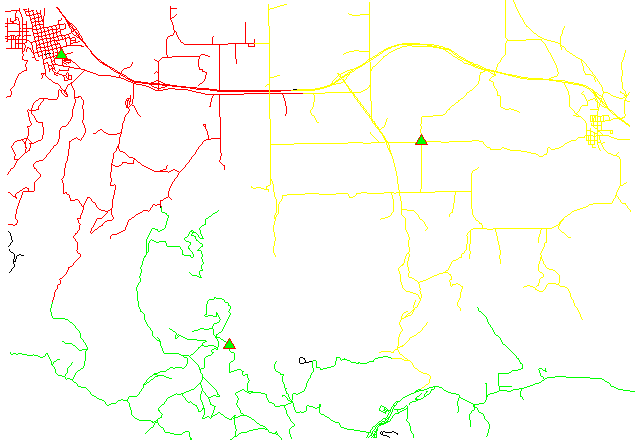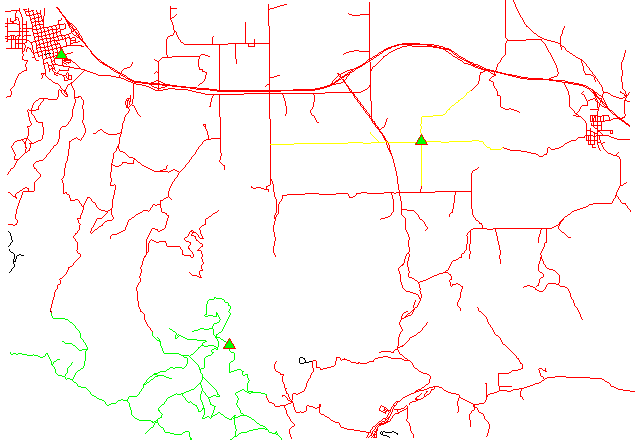Note: A new GRASS GIS stable version has been released: GRASS GIS 7. Go directly to the new manual page here

## NAME

v.net.alloc - Allocate subnets for nearest centres (direction from centre).
Centre node must be opened (costs >= 0). Costs of centre node are used in calculation

## KEYWORDS

vector, network, allocation

## SYNOPSIS

v.net.alloc
v.net.alloc help
v.net.alloc [-g] input=name output=name [type=string[,string,...]] [alayer=integer] [nlayer=integer] [afcolumn=string] [abcolumn=string] [ncolumn=string] ccats=range [--overwrite] [--verbose] [--quiet]

### Flags:

-g
Use geodesic calculation for longitude-latitude locations
--overwrite
Allow output files to overwrite existing files
--verbose
Verbose module output
--quiet
Quiet module output

### Parameters:

input=name
Name of input vector map
output=name
Name for output vector map
type=string[,string,...]
Arc type
Options: line,boundary
Default: line,boundary
alayer=integer
Layer number
Arc layer
Default: 1
nlayer=integer
Layer number
Node layer
Default: 2
afcolumn=string
Arc forward/both direction(s) cost column (number)
abcolumn=string
Arc backward direction cost column (number)
ncolumn=string
Node cost column (number)
ccats=range
Category values
Categories of centres (points on nodes) to which net will be allocated, layer for this categories is given by nlayer option

## DESCRIPTION

v.net.alloc allocates subnets for nearest centers (direction from center). center nodes must be opened (costs >= 0). Costs of center nodes are used in the calculation.

Costs may be either line lengths, or attributes saved in a database table. These attribute values are taken as costs of whole segments, not as costs to traverse a length unit (e.g. meter) of the segment. For example, if the speed limit is 100 km / h, the cost to traverse a 10 km long road segment must be calculated as
length / speed = 10 km / (100 km/h) = 0.1 h.
Supported are cost assignments for both arcs and nodes, and also different costs for both directions of a vector line. For areas, costs will be calculated along boundary lines.

The input vector needs to be prepared with v.net operation=connect in order to connect points representing center nodes to the network.

## NOTES

Nodes and arcs can be closed using cost = -1.

Center nodes can also be assigned to vector nodes using wxGUI vector digitizer.

## EXAMPLES

1. Subnetwork allocation using distance:2. Subnetwork allocation using traveling time:Example 1: Calculating subnets for 3 center nodes using distances

```# Spearfish

# center nodes:
echo "591235.5|4926306.62|1
596591.8|4917042.5|2
602722.9|4923544.2|3" | v.in.ascii in=- out=centernodes

# connect points to network

# allocate, specifying range of center cats (easier to catch all):

# report categories
```
To display the result, run for example:
```# show result
d.mon x0

# the result has to be selected by category number of the relevant node:
d.vect myroads_net_alloc cat=1 col=red layer=1
d.vect myroads_net_alloc cat=2 col=green layer=1
d.vect myroads_net_alloc cat=3 col=yellow layer=1

# center nodes
d.vect myroads_net col=red icon=basic/triangle fcol=green size=12 layer=2
```

Example 2: Calculating subnets for 3 center nodes using traveling time

```# Spearfish

# center nodes:
echo "591235.5|4926306.62|1
596591.8|4917042.5|2
602722.9|4923544.2|3" | v.in.ascii in=- out=centernodes

# create lines map connecting points to network
v.net myroads points=centernodes out=myroads_net op=connect thresh=500 alayer=1 nlayer=2

# set up costs

# create unique categories for each road in layer 3

# add new table for layer 3
v.db.addtable myroads_net_time layer=3 col="cat integer,label varchar(43),length double precision,speed double precision,cost double precision,bcost double precision"

# copy road type to layer 3
v.to.db myroads_net_time layer=3 qlayer=1 opt=query qcolumn=label columns=label

v.to.db myroads_net_time layer=3 type=line option=length col=length unit=miles

# set speed limits in miles / hour
v.db.update myroads_net_time layer=3 col=speed val="5.0"
v.db.update myroads_net_time layer=3 col=speed val="75.0" where="label='interstate'"
v.db.update myroads_net_time layer=3 col=speed val="75.0" where="label='primary highway, hard surface'"
v.db.update myroads_net_time layer=3 col=speed val="50.0" where="label='secondary highway, hard surface'"
v.db.update myroads_net_time layer=3 col=speed val="25.0" where="label='light-duty road, improved surface'"
v.db.update myroads_net_time layer=3 col=speed val="5.0" where="label='unimproved road'"

# define traveling costs as traveling time in minutes:

# set forward costs
v.db.update myroads_net_time layer=3 col=cost val="length / speed * 60"
# set backward costs
v.db.update myroads_net_time layer=3 col=bcost val="length / speed * 60"

# subnetwork allocation with fastest paths
v.net.alloc in=myroads_net_time alayer=3 nlayer=2 afcol=cost abcol=bcost out=myroads_net_alloc_time ccats=1-3
```
To display the result, run for example:
```# show result
d.mon x0
d.vect myroads_net type=line layer=1

# the result has to be selected by category number of the relevant node:
d.vect myroads_net_alloc_time cat=1 col=red layer=1
d.vect myroads_net_alloc_time cat=2 col=green layer=1
d.vect myroads_net_alloc_time cat=3 col=yellow layer=1

# center nodes
d.vect myroads_net_time col=red icon=basic/triangle fcol=green size=12 type=point layer=2
```